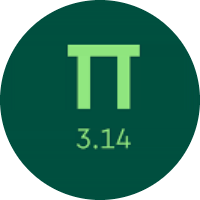# Learning the Pythagorean Theorem

In this quiz, you will get to know about the Pythagorean theorem. You will find problems on finding hypotenuse, base, and height. You will also learn about the use of the Pythagorean theorem.

Start Quiz

What is the length of the hypotenuse? If necessary, round to the nearest tenth.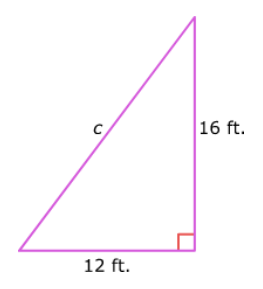20

15

16

12

What is the length of the hypotenuse? If necessary, round to the nearest tenth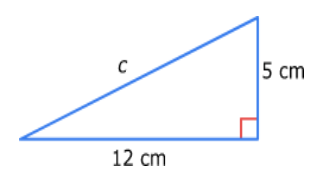25

13

17

9

What is the length of the missing leg? If necessary, round to the nearest tenth.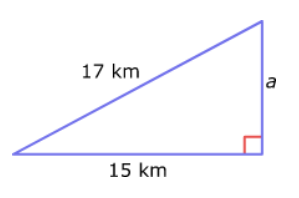6

9

4

8

What is the length of the missing leg? If necessary, round to the nearest tenth.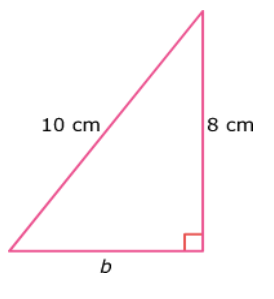6

5

3

8

What is the perimeter? If necessary, round to the nearest tenth.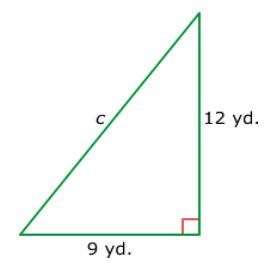36

32

45

26

What is the perimeter? If necessary, round to the nearest tenth.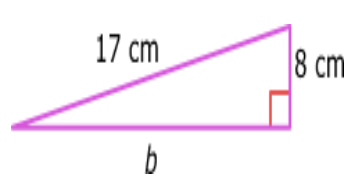34

40

45

20

Bloomington Street and Cedarburg Avenue intersect. If Bloomington Street is 5 meters wide and Cedarburg Avenue is 12 meters wide, what is the distance between two opposite corners of the intersection?

12

13

15

17

Nick just went down the slide at the playground. He walks 6 feet to get from the end of the slide back to the ladder. Then he climbs 8 feet to the top of the slide again. How long is the slide?

12 feet

13 feet

10 feet

8 feet

Quiz/Test Summary
Title: Learning the Pythagorean Theorem
Questions: 8
Contributed by: